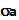## Wednesday, April 1, 2009

### Irodov Problem 1.292As the rod rotates, the centrifugal force pulls the two halves of the rod (AO and OB) apart and threatens to break the rod into two. Consider an infinitesimally small piece of the rod of length dx at a distance x from the center O. Let the surface area of cross-section of the rod be a. The mass of this piece of the rod will be dm = padx, where p is the density of the material. This mass will experience a centrifugal force of.
The total cnetrifugal force acting on OB at point O can be computed by integrating the forces acting over all infinitesimally small pieces in OB as,This force must be provided for by the stressin the rod t point O, then the force acting at point O will be. Hence, we have,Here,is the tensile strength of the material of the rod.